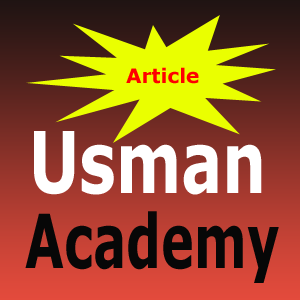# Decimal Binary Octal, Hexadecimal Conversion Practice Question for Quiz Final Exam

Number systems Decimal  Binary  Octal  Hexadecimal Conversion Practice Question for Quiz Final Exam Solutions to binary practice problems -

A) Covert the following numbers into decimal
(16.5)16    (26.24)8   (10110.0101)2

B)   Add and multiply following numbers without converting them into Decimal.
Binary Numbers 1011 and 101
C) Find the 16’s complement of the following Hexadecimal Numbers C3DF
D) Convert C3DF to Binary
E) Find the 2’s complement of the result obtained from (D)
F) Convert the answer of (E) into Hexadecimal and compare it with (C)

G) Perform Subtraction on the given Unsigned Binary Numbers Using 2’s complement:

1. 10011 – 10010           2.   101000 – 10101

H) The following decimal numbers are shown in the signed magnitude form: +9,286 and +801. Convert them to signed *10’s complement form* and perform the following operations: (Note that the sum is +10,627 and requires 5 Digits and a Sign)

1. (+9286) + (-801)         2.   (-9286) + (-801)

I) Represent the Decimal Number 6,228 in 1) BCD 2) Excess 3 Code and 2421 code

J) Decode the following ASCII Code
1010011 1110100 1100101 1110110 1100101 0100000 1001010 1101111 1100010 1110011

K) Solve problems 1.35 and 1.36 at the end of chapter 1 (Course Book) by means of a timing diagram.• Title : Decimal Binary Octal, Hexadecimal Conversion Practice Question for Quiz Final Exam
• Posted by :
• Date : 02:11
• Labels :